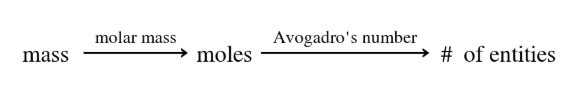# Problem: The fuel used in many disposable lighters is liquid butane, C4H10. Butane has a molecular weight of 58.1 grams in one mole. How many carbon atoms are in 1.50 g of butane? Express the number of atoms to three significant figures.

🤓 Based on our data, we think this question is relevant for Professor Santra's class at UCF.

###### FREE Expert Solution

Use the mole concept where moles we calculate moles from moles and then # of entities to from moles

Dimensional analysis will appear as:###### Problem Details

The fuel used in many disposable lighters is liquid butane, C4H10. Butane has a molecular weight of 58.1 grams in one mole. How many carbon atoms are in 1.50 g of butane? Express the number of atoms to three significant figures.

What scientific concept do you need to know in order to solve this problem?

Our tutors have indicated that to solve this problem you will need to apply the Mole Concept concept. You can view video lessons to learn Mole Concept. Or if you need more Mole Concept practice, you can also practice Mole Concept practice problems.

What is the difficulty of this problem?

Our tutors rated the difficulty ofThe fuel used in many disposable lighters is liquid butane, ...as medium difficulty.

How long does this problem take to solve?

Our expert Chemistry tutor, Dasha took 3 minutes and 36 seconds to solve this problem. You can follow their steps in the video explanation above.

What professor is this problem relevant for?

Based on our data, we think this problem is relevant for Professor Santra's class at UCF.